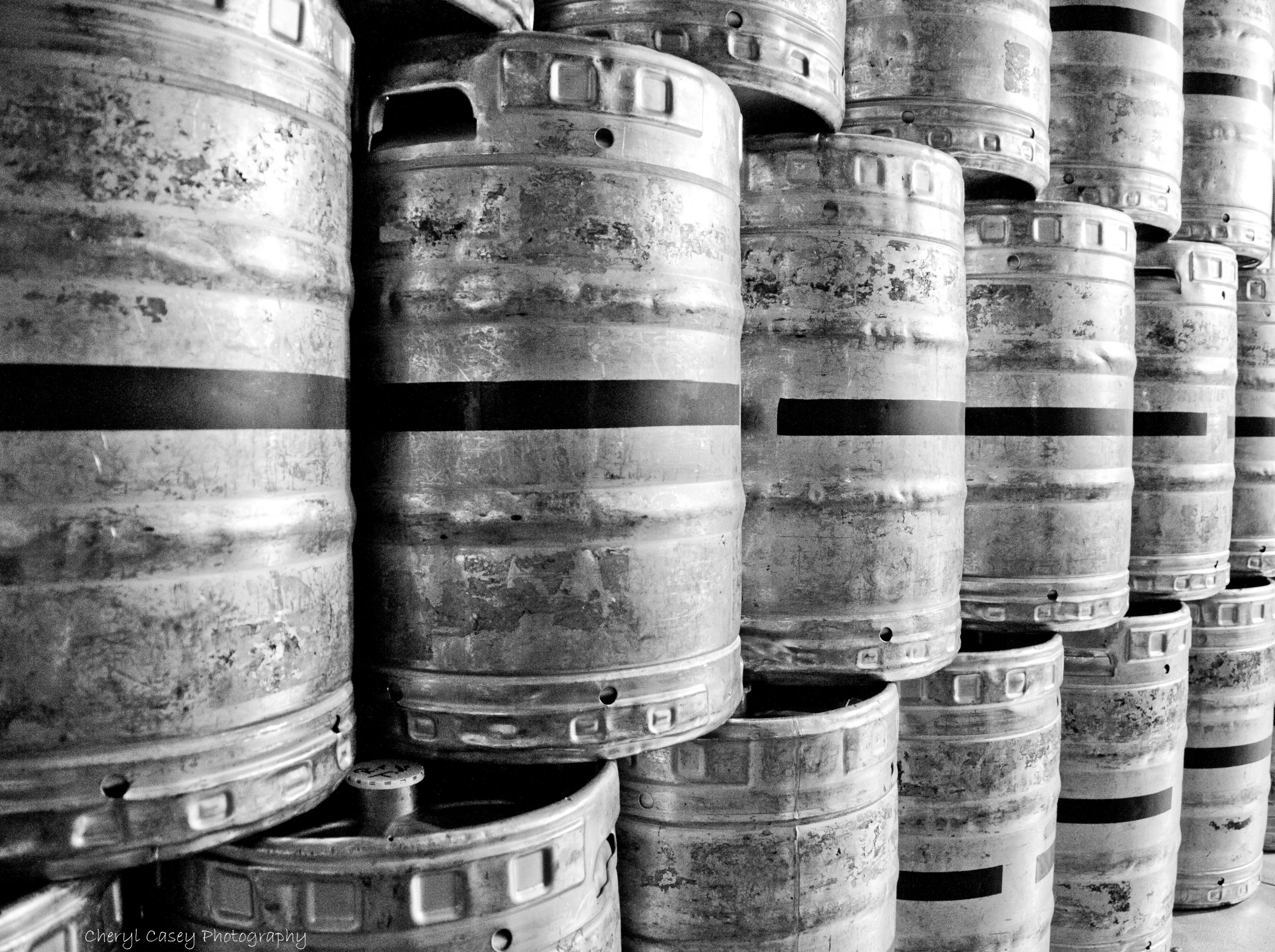## How to Weigh Draft Beer Kegs for Bar Inventory (with Free Spreadsheet)

By Stephen Ngo## Of all the ways to count kegs, using a keg scale to weigh these containers is by the far the most precise method.

But to get the most out of that keg scale, you still need an efficient liquor inventory workflow and an understanding of how to calculate your remaining product from those weight measurements. After all, knowing how to weigh a keg is one of the most useful skill every bar manager should possess.

We’ve built a free keg weight inventory spreadsheet to make this a whole lot easier. But if you still want a guide to the best keg inventory workflow—and an exploration of the calculations that our spreadsheet uses to calculate remaining product—read on.Here’s how to count kegs in inventory using a scale in five steps:

### 1. Weigh Kegs When Full

This step is relatively intuitive. To know the proportion of liquid that remains in a partially depleted keg, you must first know how much liquid is in that keg when it’s full. So weigh each keg when full and record each full keg weight.

### 2. Weigh Kegs When Empty

When you measure the weight of a keg that contains liquid, the measurement you take reflects not just the weight of the liquid, but of the keg itself. Failing to take the empty keg weight (or tare weight) into account can cause you to overestimate the amount of remaining product.

Say you have a keg that weighs 10 kilograms when empty and contains beer that weighs 15 kilograms. If you fail to subtract the weight of the keg when taking inventory, you’ll erroneously think that you have 25 kilograms of product (67% more than the 15 kilograms that you actually have). As a result, you’ll run out of beer earlier than you expect.

To avoid this, weigh each keg when empty. (We’ll show how to use this when you calculate your remaining inventory in Step 4.)

### 3. Weigh Kegs During Inventory

Weigh each partially full keg, and record that partially full keg weight (often referred to as gross weight) alongside the full keg weight and empty keg weight of that same keg. This step is exactly what you’d expect.

Kegs that are completely full or empty, of course, count respectively as either one full container of product, or depleted product.

### 4. Calculate Remaining Volume in Each Keg

Here comes the geeky part.

To calculate the proportion of product that remains in each partially full keg, first take the partially full keg weight minus the empty keg weight. Then divide this by the full keg weight minus the empty keg weight:

% Product Remaining =
(Partially Full Keg Weight – Empty Keg Weight)
/ (Full Keg Weight – Empty Keg Weight)

Take the example from earlier. You have a keg that weighs 10 kilograms when empty, which contains 15 kilograms of beer (for a partially full keg weight of 25 kilograms). Say that keg weighs 30 kilograms when full.

% Product Remaining =
(25kg – 10kg) / (30kg – 10kg)
= (15kg) / (20kg)
= 75% of Product Remaining

In this example, a full keg contains 20 kilograms of beer once you subtract the weight of the keg itself. 15 kilograms remain. Divide 15 kilograms by 20 kilograms.

That means the keg is 75% full.

### 5. Log Inventory Totals in a System of Record

Now that you’ve calculated the amount of each product that you have in stock, it’s vital that you record your inventory totals in a robust system of record. Whether that’s a bar inventory spreadsheet or cloud-based bar management software, maintaining consistent inventory procedures and records is vital for:

Start a FREE Trial Today! BevSpot offers full product education and account setup for all customers!

Schedule 15mins to chat with a product specialistNo comments yet... Be the first?